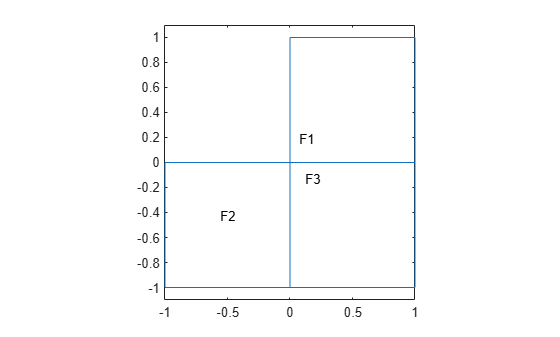Documentation

# findThermalProperties

Find thermal material properties assigned to a geometric region

## Syntax

``tmpa = findThermalProperties(thermalmodel.MaterialProperties,RegionType,RegionID)``

## Description

example

````tmpa = findThermalProperties(thermalmodel.MaterialProperties,RegionType,RegionID)` returns thermal material properties `tmpa` assigned to the specified region.```

## Examples

collapse all

Create a transient thermal model that has three faces.

```thermalmodel = createpde('thermal','transient'); geometryFromEdges(thermalmodel,@lshapeg); pdegplot(thermalmodel,'FaceLabels','on') ylim([-1.1,1.1]) axis equal```For face 1, specify the following thermal properties:

• Thermal conductivity is 10 W/(m*C)

• Mass density is 1 kg/m^3

• Specific heat is 0.1 J/(kg*C)

```thermalProperties(thermalmodel,'ThermalConductivity',10,... 'MassDensity',1,... 'SpecificHeat',0.1,... 'Face',1);```

For face 2, specify the following thermal properties:

• Thermal conductivity is 20 W/(m*C)

• Mass density is 2 kg/m^3

• Specific heat is 0.2 J/(kg*C)

```thermalProperties(thermalmodel,'ThermalConductivity',20,... 'MassDensity',2,... 'SpecificHeat',0.2,... 'Face',2);```

For face 1, specify the following thermal properties: thermal conductivity is 30 W/(m*C), mass density is 3 kg/m^3, specific heat is 0.3 J/(kg*C).

• Thermal conductivity is 30 W/(m*C)

• Mass density is 3 kg/m^3

• Specific heat is 0.3 J/(kg*C)

```thermalProperties(thermalmodel,'ThermalConductivity',30,... 'MassDensity',3,... 'SpecificHeat',0.3,... 'Face',3);```

Check the material properties specification for face 1.

`mpaFace1 = findThermalProperties(thermalmodel.MaterialProperties,'Face',1)`
```mpaFace1 = ThermalMaterialAssignment with properties: RegionType: 'face' RegionID: 1 ThermalConductivity: 10 MassDensity: 1 SpecificHeat: 0.1000 ```

Check the heat source specification for faces 2 and 3.

```mpa = findThermalProperties(thermalmodel.MaterialProperties,'Face',[2,3]); mpaFace2 = mpa(1)```
```mpaFace2 = ThermalMaterialAssignment with properties: RegionType: 'face' RegionID: 2 ThermalConductivity: 20 MassDensity: 2 SpecificHeat: 0.2000 ```
`mpaFace3 = mpa(2)`
```mpaFace3 = ThermalMaterialAssignment with properties: RegionType: 'face' RegionID: 3 ThermalConductivity: 30 MassDensity: 3 SpecificHeat: 0.3000 ```

Create a geometry that consists of three stacked cylinders and include the geometry in a thermal model.

`gm = multicylinder(10,[1 2 3],'ZOffset',[0 1 3])`
```gm = DiscreteGeometry with properties: NumCells: 3 NumFaces: 7 NumEdges: 4 NumVertices: 4 ```
```thermalmodel = createpde('thermal'); thermalmodel.Geometry = gm; pdegplot(thermalmodel,'CellLabels','on','FaceAlpha',0.5)```Thermal conductivity of the cylinder `C1` is 10 W/(m*C).

`thermalProperties(thermalmodel,'ThermalConductivity',10,'Cell',1);`

Thermal conductivity of the cylinder `C2` is 20 W/(m*C).

`thermalProperties(thermalmodel,'ThermalConductivity',20,'Cell',2);`

Thermal conductivity of the cylinder `C3` is 30 W/(m*C).

`thermalProperties(thermalmodel,'ThermalConductivity',30,'Cell',3);`

Check the material properties specification for cell 1:

`mpaCell1 = findThermalProperties(thermalmodel.MaterialProperties,'Cell',1)`
```mpaCell1 = ThermalMaterialAssignment with properties: RegionType: 'cell' RegionID: 1 ThermalConductivity: 10 MassDensity: [] SpecificHeat: [] ```

Check the heat source specification for cells 2 and 3:

```mpa = findThermalProperties(thermalmodel.MaterialProperties,'Cell',2:3); mpaCell2 = mpa(1)```
```mpaCell2 = ThermalMaterialAssignment with properties: RegionType: 'cell' RegionID: 2 ThermalConductivity: 20 MassDensity: [] SpecificHeat: [] ```
`mpaCell3 = mpa(2)`
```mpaCell3 = ThermalMaterialAssignment with properties: RegionType: 'cell' RegionID: 3 ThermalConductivity: 30 MassDensity: [] SpecificHeat: [] ```

## Input Arguments

collapse all

Material properties of the model, specified as the `MaterialProperties` property of a thermal model.

Example: `thermalmodel.MaterialProperties`

Geometric region type, specified as `'Face'` or `'Cell'`.

Example: `findThermalProperties(thermalmodel.MaterialProperties,'Cell',1)`

Data Types: `char` | `string`

Geometric region ID, specified as a vector of positive integers. Find the region IDs using `pdegplot`, as shown in Create Geometry and Remove Face Boundaries or STL File Import.

Example: `findThermalProperties(thermalmodel.MaterialProperties,'Face',1:3)`

Data Types: `double`

## Output Arguments

collapse all

Material properties assignment, returned as a ThermalMaterialAssignment Properties object.

## See Also

#### Introduced in R2017a

##### SupportGet trial now